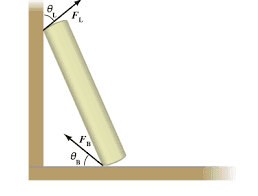# A book rests at an angle against one side of a bookshelf...

Homework Statement:
A 0.135 kg
book rests at an angle against one side of a bookshelf. The magnitude and direction of the total force exerted on the book by the left side of the bookshelf are given by

|𝐹L|=0.575 N𝜃L=55.0°

What must the magnitude |𝐹B|
and direction 𝜃B
of the total force exerted on the book by the bottom of the bookshelf be in order for the book to remain in this position?
Relevant Equations:
Fnet=ma1.52cos(31)+Fbsin(Θb) = (0.305)(9.8)
Fbsin(Θb) = 1.69

Now for horizontal direction:
Fbcos(Θb) = FLsin(ΘL)
cos(Θb) = FLsin(ΘL)/Fb
cos(Θb) = (1.52 x sin31)/1.69

cosΘb=0.464
Θb = 62.35

I thought to find Fb I would just plug the Θb value into one of the trig functions, but apparently both of my answers are not right. What am I doing wrong?

Homework Helper
Gold Member
It would help us help you if you used symbols instead of numbers. Failing that, at least you could tell us where the numbers you are using come from. For example, your first equation
1.52cos(31)+Fbsin(Θb) = (0.305)(9.8)
is totally mysterious to me.
Where did the force of 1.52, the angle of 31 and the mass of 0.305 come from? Put yourself in our position. We cannot help you find what you are doing wrong if we don't know what you are doing.

•jbriggs444 and erobz
•